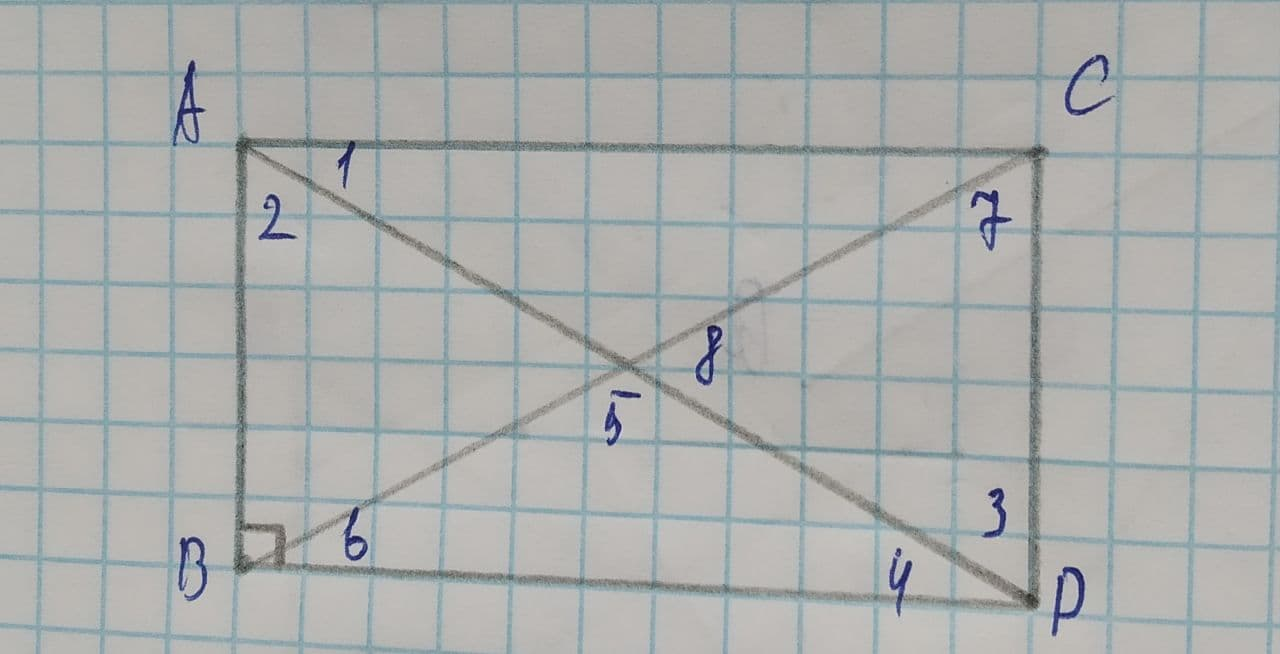# Determine the measure of angle 6Yulia 2021-07-11 Answered
To determine:The measure of angle 6.
Given:
ABDC is a rectangle.$m\mathrm{\angle }1=38$
You can still ask an expert for help

## Want to know more about Congruence?

• Questions are typically answered in as fast as 30 minutes

Solve your problem for the price of one coffee

• Math expert for every subject
• Pay only if we can solve itgotovub

Calculation:
The diagonals of a rectangle are congruent and also bisect each other.
So, triangle AEC is an isosceles triangle.
According to Isosceles Triangle Theorem, $\mathrm{\angle }1\stackrel{\sim }{=}\mathrm{\angle }ACB$.
Also, according to the definition of a rectangle, $\stackrel{―}{AC}\parallel \stackrel{―}{BD}$.
Using Alternate Interior Angles Theorem. $\mathrm{\angle }6\stackrel{\sim }{=}\mathrm{\angle }ACB$.
By Transitive Property of Congruence. $\mathrm{\angle }6\stackrel{\sim }{=}\mathrm{\angle }1$.
So,
$m\mathrm{\angle }6=m\mathrm{\angle }1$
$m\mathrm{\angle }6=38$